#2018 iT 邦幫忙鐵人賽DAY 22
2
Data Technology

## 【22】當老闆疑惑地問：所以我可以在這個網頁調整參數，那有哪些參數可以調？

``````fluidRow(
column(4,
div(style = "background-color: GreenYellow;",
h4("黃綠區塊")
)
),
column(4,
div(style = "background-color: Coral;",
h4("橘色區塊")
)
),
column(4,
div(style = "background-color: LightBlue;",
h4("淡藍區塊")
)
)
)
``````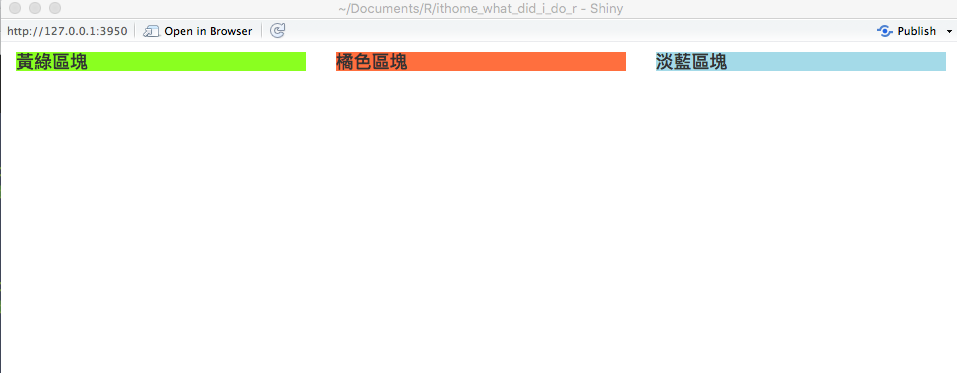``````fluidRow(
column(6,
div(style = "background-color: GreenYellow;",
h4("黃綠區塊")
)
),
column(3,
div(style = "background-color: Coral;",
h4("橘色區塊")
)
),
column(3,
div(style = "background-color: LightBlue;",
h4("淡藍區塊")
)
)
)
``````1. sliderInput

``````sliderInput('sampleSize', 'Sample Size',
min=10, max=1000, value=500,
step=10, round=0)
``````

min和max 分別是bar的最小和最大值， value是預設值，step是刻度，round是上面的值是否要四捨五入。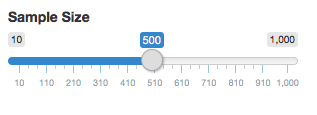1. checkboxInput

``````checkboxInput('check1', '勾選按鈕1')
``````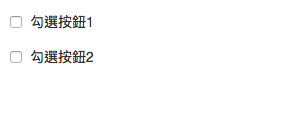1. selectInput

``````selectInput('select1', '選擇1', c("A", "B", "C"))
``````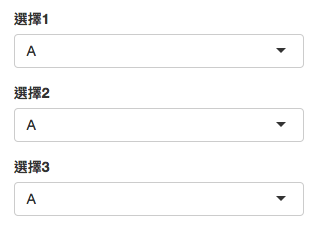4.numericInput

``````numericInput("num", h3("數字選擇"), value = 1)
``````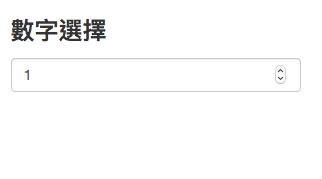5.actionButton

``````actionButton("button", "按鈕")
``````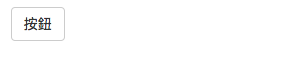1. dateInput
日期選擇器，點擊後會打開瀏覽器內建的日期選擇。
``````dateInput("date", h3("Date input")
``````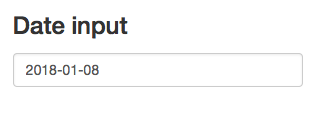7.textInput

``````textInput("text", h3("Text input"), value = "Enter text...")
``````ref
day22原始碼